# How to set, clear and toggle a single bit of a number in JavaScript?

Given a JavaScript number and the task is to set, clear and toggle a single bit of any number. Here are few approaches discussed with the help of JavaScript.

1. Setting the bit:
• Get the position of the bit to be set(starts from right, first bit is at 0 position), Ex. (bitSet = 3)
• Get the mask by mask = 1 << setBit, this mask is helpful in setting, clearing as well in toggling the bit.
• Use OR operator to set the particular bit.

Example: This example uses the approach discussed above.

 ` ` `<``html``> ` ` `  `<``head``> ` `    ``<``title``> ` `        ``Set, clear and toggle a single bit of a number. ` `    `` ` ` ` ` `  `<``body` `style``=``"text-align:center;"``> ` `    ``<``h1` `style``=``"color: green"``>   ` `            ``GeeksForGeeks   ` `        `` ` `    ``<``p` `id``=``"GFG_UP"` `style``=``"font-size: 20px; font-weight: bold;"``> ` `    `` ` `    ``<``button` `onclick``=``"gfg_Run()"``> ` `        ``Click Here ` `    `` ` `    ``<``p` `id``=``"GFG_DOWN"` `style``=``"color:green;"``> ` `    `` ` `    ``<``script``> ` `        ``var el_up = document.getElementById("GFG_UP"); ` `        ``var el_down = document.getElementById("GFG_DOWN"); ` `        ``var n = 6; ` `        ``var setBit = 3; ` `        ``el_up.innerHTML = "Click on the button to set the " ` `        ``+ setBit +  ` `          ``" bit of the number.<``br``>Number - " + n ` ` `  `        ``function gfg_Run() { ` `            ``var mask = 1 << ``setBit``; ` `            ``n` `= n | mask; ` `            ``el_down.innerHTML` `= n; ` `        ``} ` `    `` ` ` ` ` `  ` `

Output:

• Before clicking on the button: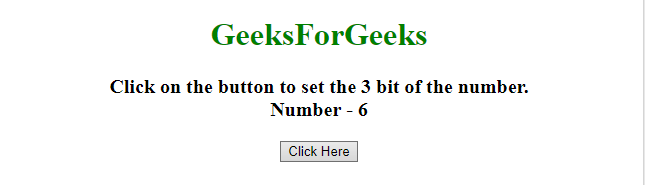• After clicking on the button: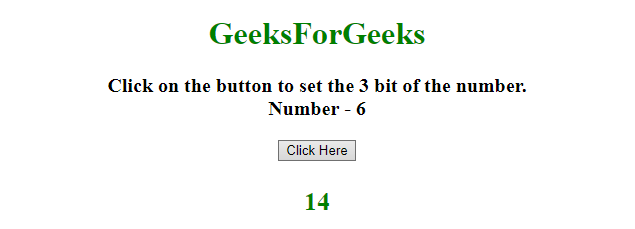2. Clearing the bit:
• Get the position of the bit to be clear(starts from right, first bit is at 0 position), Ex. (bitSet = 3)
• Use AND operator with negation of mask to clear the particular bit.

Example: This example uses the approach discussed above.

 ` ` `<``html``> ` ` `  `<``head``> ` `    ``<``title``> ` `        ``Set, clear and toggle a single bit of a number. ` `    `` ` ` ` ` `  `<``body` `style``=``"text-align:center;"``> ` `    ``<``h1` `style``=``"color: green"``>   ` `            ``GeeksForGeeks   ` `        `` ` `    ``<``p` `id``=``"GFG_UP"``> ` `    `` ` `    ``<``button` `onclick``=``"gfg_Run()"``> ` `        ``Click Here ` `    `` ` `    ``<``p` `id``=``"GFG_DOWN"` `style``=``"color:green;"``> ` `    `` ` `    ``<``script``> ` `        ``var el_up = document.getElementById("GFG_UP"); ` `        ``var el_down = document.getElementById("GFG_DOWN"); ` `        ``var n = 6; ` `        ``var setBit = 2; ` `        ``el_up.innerHTML = ` `          ``"Click on the button to clear the "  ` `        ``+ setBit + ` `          ``" bit of the number.<``br``>Number - " + n ` ` `  `        ``function gfg_Run() { ` `            ``var mask = 1 << ``setBit``; ` `            ``n &= ~mask; ` `            ``el_down.innerHTML` `= n; ` `        ``} ` `    `` ` ` ` ` `  ` `

Output:

• Before clicking on the button: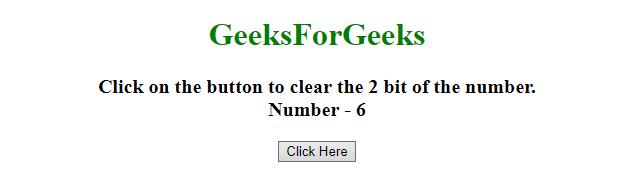• After clicking on the button: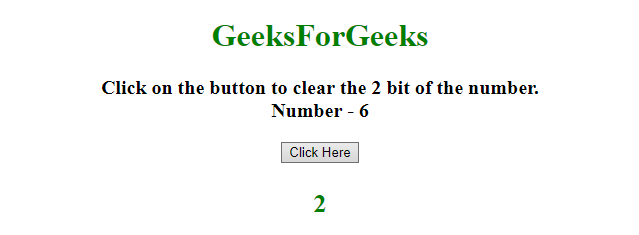3. Toggling the bit:
• Get the position of the bit to be toggle(starts from right, first bit is at 0 position), Ex. (bitSet = 3)
• Use XOR operator to toggle the particular bit.

Example: This example uses the approach discussed above.

 ` ` `<``html``> ` ` `  `<``head``> ` `    ``<``title``> ` `        ``Set, clear and toggle a single bit of a number. ` `    `` ` ` ` ` `  `<``body` `style``=``"text-align:center;"``> ` `    ``<``h1` `style``=``"color: green"``>   ` `            ``GeeksForGeeks   ` `        `` ` `    ``<``p` `id``=``"GFG_UP"``> ` `    `` ` `    ``<``button` `onclick``=``"gfg_Run()"``> ` `        ``Click Here ` `    `` ` `    ``<``p` `id``=``"GFG_DOWN"` `style``=``"color:green;"``> ` `    `` ` `    ``<``script``> ` `        ``var el_up = document.getElementById("GFG_UP"); ` `        ``var el_down = document.getElementById("GFG_DOWN"); ` `        ``var n = 6; ` `        ``var setBit = 3; ` `        ``el_up.innerHTML =  ` `          ``"Click on the button to toggle the "  ` `        ``+ setBit +  ` `          ``" bit of the number.<``br``>Number - " + n ` ` `  `        ``function gfg_Run() { ` `            ``var mask = 1 << ``setBit``; ` `            ``n ^= mask; ` `            ``el_down.innerHTML` `= n; ` `        ``} ` `    `` ` ` ` ` `  ` `

Output:

• Before clicking on the button: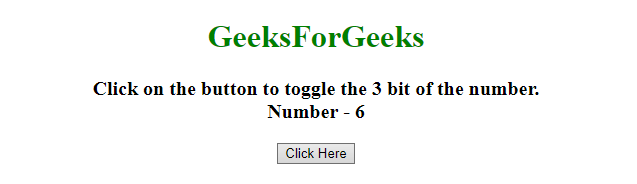• After clicking on the button: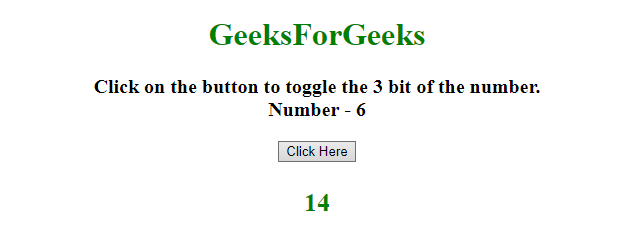My Personal Notes arrow_drop_upCheck out this Author's contributed articles.

If you like GeeksforGeeks and would like to contribute, you can also write an article using contribute.geeksforgeeks.org or mail your article to contribute@geeksforgeeks.org. See your article appearing on the GeeksforGeeks main page and help other Geeks.

Please Improve this article if you find anything incorrect by clicking on the "Improve Article" button below.

Article Tags :

Be the First to upvote.

Please write to us at contribute@geeksforgeeks.org to report any issue with the above content.